# Search by Topic

Filter by: Content type:
Age range:
Challenge level:

### There are 9 results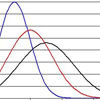### Into the Normal Distribution

##### Age 16 to 18 Challenge Level:

Investigate the normal distribution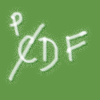### PCDF

##### Age 16 to 18 Challenge Level:

When can a pdf and a cdf coincide?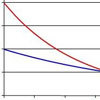### Into the Exponential Distribution

##### Age 16 to 18 Challenge Level:

Get into the exponential distribution through an exploration of its pdf.### Scale Invariance

##### Age 16 to 18 Challenge Level:

By exploring the concept of scale invariance, find the probability that a random piece of real data begins with a 1.### Pdf Stories

##### Age 16 to 18 Challenge Level:

Invent scenarios which would give rise to these probability density functions.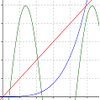### Exponential Intersection

##### Age 16 to 18 Challenge Level:

Can the pdfs and cdfs of an exponential distribution intersect?### Circle Pdf

##### Age 16 to 18 Challenge Level:

What happens if this pdf is the arc of a circle?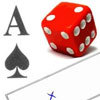### Distribution Differences

##### Age 14 to 16 Challenge Level:

How could you compare different situation where something random happens ? What sort of things might be the same ? What might be different ?### PDF

##### Age 16 to 18 Challenge Level:

Given a probability density function find the mean, median and mode of the distribution.